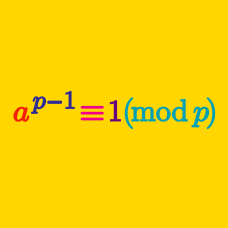Number Theory

# Basic Applications of Modular Arithmetic: Level 4 Challenges

Find the remainder when $70!$ is divided by $5183$.

Note: Don't use a computational device!

What is the sum of all six-digit positive integer(s) such that they satisfy the property that the number equals to the last six (rightmost) digits of its own square?

$\large \displaystyle\sum_{k=0}^{1007!+1}{10^k}$

Find the remainder when the summation above is divided by the summation below.

$\large \displaystyle\sum_{k=0}^{1008}{10^k}$

Find the largest $n<10,000$ such that $\displaystyle \prod_{k=0}^{n} \binom{n}{k}$ is an odd number.

$\Huge 6^{6^{6^{6^{6^6}}}}$

Find the $6^\text{th}$ last digit from the right of the decimal representation of the above number.

×